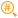# Parse Number

 Block Group: Number Formatting Icon:The Parse Number block returns the first number that it can parse from a string. If the input string contains more than one numeric value, only the first value is extracted. If the input string does not contain any valid numeric values, a NaN error is returned.

For information on using dataflow blocks, see Dataflow.

For accepted and excluded arguments of Number Formatting blocks, see Number Formatting.

## Input/Output Property

The following property of the Parse Number block can take input and give output.

• input (string)

input specifies the string from which to extract a numeric value.

## Output Property

The following property of the Parse Number block can give output but cannot take input.

• output (number)

output returns the first numeric value parsed from the input string.

## Examples

The following image shows two examples of the Parse Number block. The topmost Parse Number block extracts only the number from a string that also includes a unit label. The bottommost Parse Number block extracts the first number from a text string that includes multiple numbers.